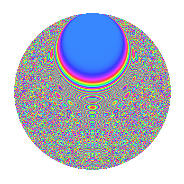# Properties

 Label 3234.2.bhLevel 3234 Weight 2 Character orbit bh Rep. character $$\chi_{3234}(331,\cdot)$$ Character field $$\Q(\zeta_{21})$$ Dimension 1104 Sturm bound 1344

# Related objects

## Defining parameters

 Level: $$N$$ $$=$$ $$3234 = 2 \cdot 3 \cdot 7^{2} \cdot 11$$ Weight: $$k$$ $$=$$ $$2$$ Character orbit: $$[\chi]$$ $$=$$ 3234.bh (of order $$21$$ and degree $$12$$) Character conductor: $$\operatorname{cond}(\chi)$$ $$=$$ $$49$$ Character field: $$\Q(\zeta_{21})$$ Sturm bound: $$1344$$

## Dimensions

The following table gives the dimensions of various subspaces of $$M_{2}(3234, [\chi])$$.

Total New Old
Modular forms 8160 1104 7056
Cusp forms 7968 1104 6864
Eisenstein series 192 0 192

## Trace form

 $$1104q + 92q^{4} + 92q^{9} + O(q^{10})$$ $$1104q + 92q^{4} + 92q^{9} + 92q^{16} - 8q^{19} + 8q^{21} + 84q^{25} + 32q^{29} + 4q^{33} - 24q^{34} + 32q^{35} - 184q^{36} - 44q^{37} - 16q^{38} + 64q^{39} + 160q^{41} + 48q^{42} + 104q^{47} + 24q^{49} + 32q^{50} + 104q^{53} - 32q^{57} + 52q^{58} + 208q^{59} + 104q^{61} - 184q^{64} + 8q^{66} - 24q^{69} + 40q^{70} + 32q^{71} - 16q^{73} - 8q^{75} + 16q^{76} + 8q^{77} - 80q^{78} + 8q^{79} + 92q^{81} + 16q^{82} + 192q^{83} - 16q^{84} - 16q^{86} - 24q^{87} - 40q^{89} + 248q^{91} + 16q^{93} - 16q^{94} - 32q^{95} + 104q^{97} + O(q^{100})$$

## Decomposition of $$S_{2}^{\mathrm{new}}(3234, [\chi])$$ into newform subspaces

The newforms in this space have not yet been added to the LMFDB.

## Decomposition of $$S_{2}^{\mathrm{old}}(3234, [\chi])$$ into lower level spaces

$$S_{2}^{\mathrm{old}}(3234, [\chi]) \cong$$ $$S_{2}^{\mathrm{new}}(49, [\chi])$$$$^{\oplus 8}$$$$\oplus$$$$S_{2}^{\mathrm{new}}(98, [\chi])$$$$^{\oplus 4}$$$$\oplus$$$$S_{2}^{\mathrm{new}}(147, [\chi])$$$$^{\oplus 4}$$$$\oplus$$$$S_{2}^{\mathrm{new}}(294, [\chi])$$$$^{\oplus 2}$$$$\oplus$$$$S_{2}^{\mathrm{new}}(539, [\chi])$$$$^{\oplus 4}$$$$\oplus$$$$S_{2}^{\mathrm{new}}(1078, [\chi])$$$$^{\oplus 2}$$$$\oplus$$$$S_{2}^{\mathrm{new}}(1617, [\chi])$$$$^{\oplus 2}$$

## Hecke characteristic polynomials

There are no characteristic polynomials of Hecke operators in the database